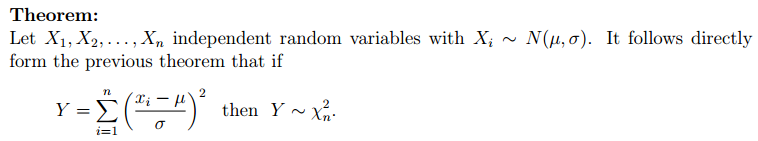Sum of K of identically distributed Independent random variables Xi,  Y=sum(xi^2) variables will be  chi squared with K degree of freedom

It can be shown that the sum of K of similarly distributed random variables Xi,  Y=sum(xi^2) variables will bepdf(y)=\frac{1}{ 2^{(k/2)} \Gamma (k/2) } y^{(\frac {k}{2}-1)}{e^{ – \frac  {y }{2} }}

Which we call chi squared with K degree of freedom

mean of k/2*2=k

Variance of K/2*4=2kThis means as k goes to infinity it becomes bell shaped N(k,2k)

based on definition of z we havehttp://en.wikipedia.org/wiki/Chi-squared_distribution

http://www.stat.ucla.edu/~nchristo/statistics100B/

9. Distributions related to normal (chi square, t, F).